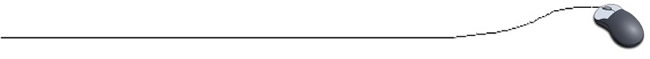While Loop Fragments

 // To print a horizontal row of 50 asterisks:  (NOTE: there are various ways of accomplishing the same task) int number = 0;  while (number < 50) {      System.out.print( "*");      number++; } System.out.println("\n Here are " + number +" stars"); int number = 1; while (number <= 50) {      System.out.print( "*");       number++; } System.out.println("\nHere are " + number + " stars");

 // To print the numbers 4 - 14 on a single line: int num = 4; while (num < 15) {      System.out.print( (num++) + " "); } int num = 4;  while (num <=14) {      System.out.print(( num++) + " "); }